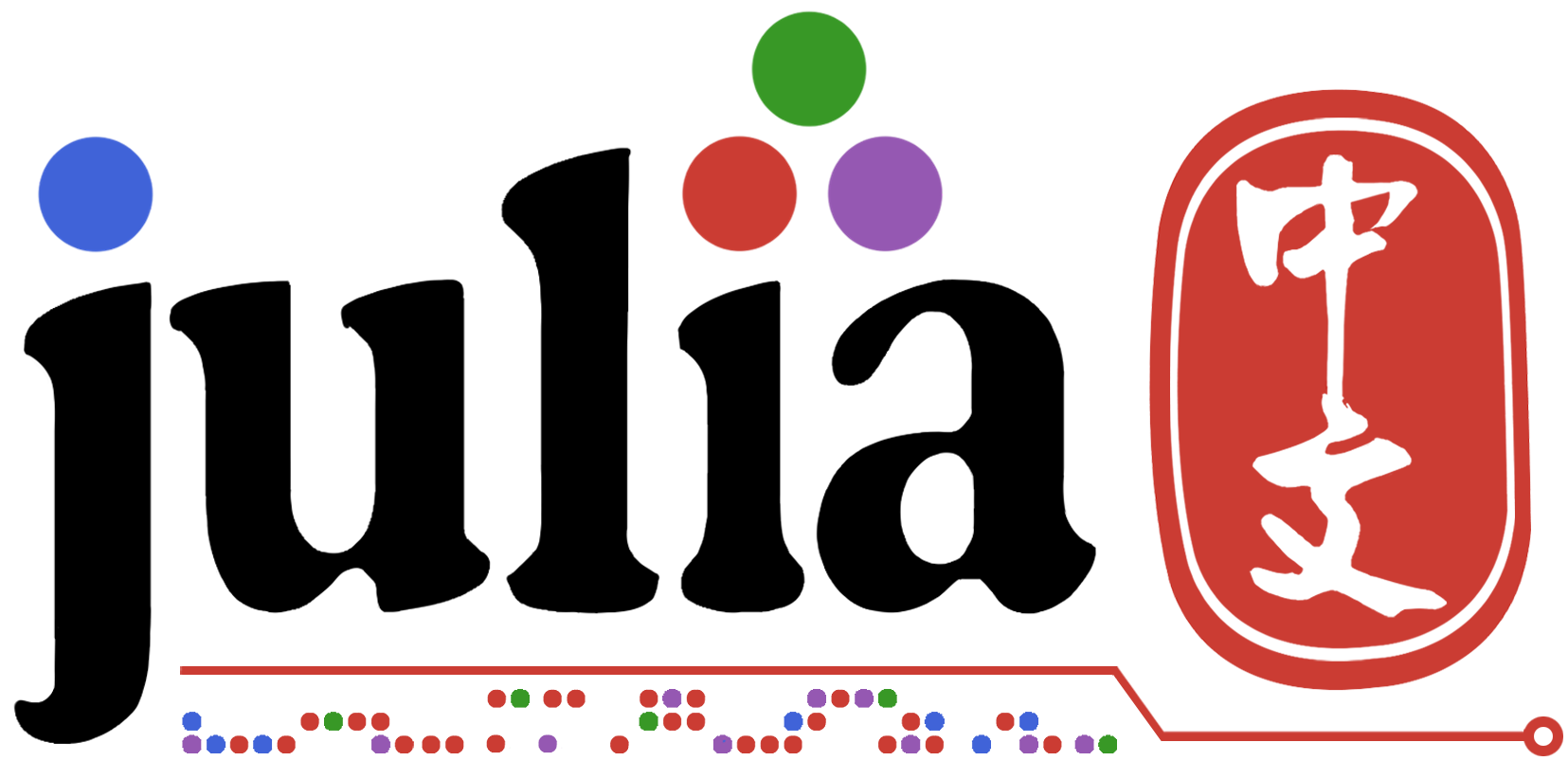# 起因

Julia 函数参数遵循有时称为 “pass-by-sharing” 的约定，这意味着变量在被传递给函数时其值并不会被复制。函数参数本身充当新的变量绑定（指向变量值的新地址），它们所指向的值与所传递变量的值完全相同。调用者可以看到对函数内可变值（如数组）的修改。这与 Scheme，大多数 Lisps，Python，Ruby 和 Perl 以及其他动态语言中的行为相同。

# 举例

``````x=reshape(collect(1:9),3,3)
function f(x)
x=x^2
end
println(f(x)) #[30 66 102; 36 81 126; 42 96 150]
println(x)  #[1 4 7; 2 5 8; 3 6 9]
``````

``````x=reshape(collect(1:9),3,3)
function g(x)
x.=x^2
end
println(g(x)) #[30 66 102; 36 81 126; 42 96 150]
println(x) #[30 66 102; 36 81 126; 42 96 150]
``````

``````y=x^2
for i in eachindex(x)
x[i]=y[i] # 这里按位置修改了 x的值。
end
``````

1赞